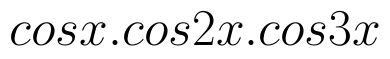Select Page

# Solutions for MCQ CBSE 12 Science Maths Relations and Functions in English

Solutions for MCQ CBSE 12 Science Maths Relations and Functions in English to enable students to get Solutions in a narrative video format for the specific question.

Expert Teacher provides Solutions for MCQ CBSE 12 Science Maths Relations and Functions through Video Solutions in English language. This video solution will be useful for students to understand how to write an answer in exam in order to score more marks. This teacher uses a narrative style for a question from Relations and Functions not only to explain the proper method of answering question, but deriving right answer too.

Please find the question below and view the Solution in a narrative video format.

Question:

Solution Video in English:

You can select video Solutions from other languages also. Please check Solutions in ( Hindi )

## Similar Questions from CBSE, 12th Science, Maths, Relations and Functions

Question 1 : The law a + b = b + a is called ______. (View Answer Video)

Question 2 : The number of binary operations which can be defined on the setis, (View Answer Video)

Question 3 : Number of binary operations on the set { a, b} are: (View Answer Video)

Question 4 : A functiondefined asis, (View Answer Video)

Question 5 :  * is a binary operation on Z such that: a * b = a + b + ab. The solution of (3* 4) *x = – 1 is,

### Application of Derivatives

Question 1 : Find the maximum value of inthe interval [1,3]. find the maximum value of the same function in [-3,-1]. (View Answer Video)

Question 2 : On which of the following intervals in the functionstrictly decreasing? (View Answer Video)

Question 3 : The volume of a cube is increasing at the rate of 9 cubic centimetres per second. How fast is the surface area increasing when the length of an edge is 10 centimetres? (View Answer Video)

Question 4 : The function f is a differentiable function and satisfies the functional equation f(x) + f(y) = f(x + y) – xy – 1for every pair x, y of real numbers. If f(1) = 1, then the number of integers n ≠ 1 for which f(n) = n is_______________. (View Answer Video)

Question 5 :

The total revenue in Rupees received from the sale of 'x' units of a product is given by :The marginal revenue, when x=15 is :

### Continuity and Differentiability

Question 1 : Differentiate the function w.r.t.x. (View Answer Video)

Question 2 : Differentiate the functionwith respect to x. (View Answer Video)

Question 3 : Differentiate the function w.r.t.x. (View Answer Video)

Question 4 : Differentiate the function w.r.t.x. (View Answer Video)

Question 5 : Find the value of k, if the area of the triangle is 4 sq unit and vertices are (-2, 0), (0, 4), (0, k). (View Answer Video)

### Inverse Trigonometric Functions

Question 1 : Evaluate :(View Answer Video)

Question 2 : Write the principal value of(View Answer Video)

Question 3 : Write in the simplest form:(View Answer Video)

Question 4 : Find the value ofWhat value do you learn from it ? (View Answer Video)

Question 5 : Evaluate :(View Answer Video)